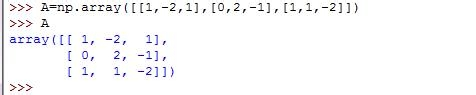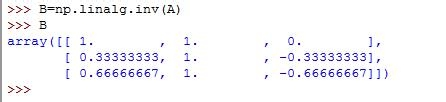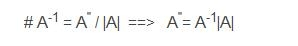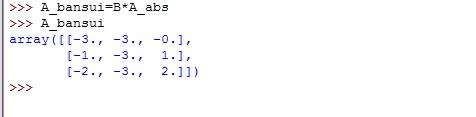• 先来求一下矩阵的逆，先引入numpy• 然后创建一个方阵A• 使用linalg.det求得方阵的行列式• 使用linalg.inv求得方阵A的逆矩阵• 接着我们利用公式：
numpy的计算方法：• 以下是今天用到的所有代码

• import numpy as np

• A=np.array([[1,-2,1],[0,2,-1],[1,1,-2]])

• A

• array([[ 1, -2, 1],

• [ 0, 2, -1],

• [ 1, 1, -2]])

• A_abs=np.linalg.det(A)

• A_abs

• -3.0000000000000004

• B=np.linalg.inv(A)

• B

• array([[ 1. , 1. , 0. ],

• [ 0.33333333, 1. , -0.33333333],

• [ 0.66666667, 1. , -0.66666667]])

• A_ni=B*A_abs

• A_ni

• array([[-3., -3., -0.],

• [-1., -3., 1.],

• [-2., -3., 2.]])

• A_bansui=B*A_abs

• A_bansui

• array([[-3., -3., -0.],

• [-1., -3., 1.],

• [-2., -3., 2.]])

### 赞助